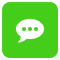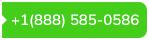×Managerial Economics

NPV

Year                            0          1                      2                      3                      4                  5

Cash flow               (20,000)    5,000                  5,000            5,000                5,000             5,000

Depreciation                           (4,000)              (4,000)           (4,000)             (4,000)           (4,000)

1,000                    1,000                   1,000                     1,000                  1,000

Tax  (at 40%)                            400                       400                       400                       400                  400

After tax income                         600                       600                       600                       600                  600

Add depreciation                        4,000                    4,000                    4,000                    4,000              4,000

Operating income                  4,600                       4,600                 4,600                        4,600             4,600

PV factor at 12%          1.000     0.8929                0.7972               0.7119                     0.6355           0.5674

Present value              (20,000)   4,107                3667.12              3,274.74                  2923.3          2610

NPV=  16582.16-20,000  = \$(3,417.84)

Internal rate of return =               average profit

Average investment

Average profit = \$ 600

Average investment

Year                1                      2                      3                      4                      5                      average

Starting           20,000         16000                  12000               8000                 4000

Depreciation     4000             4000                  4000                 4000                 4000

Ending             16000           12000                 8000                 4000                    0

Average           18000           15000                  10000               6000                  2000               10,200

IRR= 600/10200*100             = 5.88%

The project should be rejected as it gives a negative NPV and its internal rate of return is lower than the firm’s cost of capital.

No. 5

Net present value

Year                            0                      1                      2                      3                      4

Incremental returns (800,000)                     450,000             350,000               275,000             200,000

Less depreciation                                 (200,000)          (200,000)             (200,000)          (200,000)

250,000            150,000               75,000              0

Tax at 40%                                      (100,000)         (60,000)                (30,000)           0

After tax returns                                    150,000             90,000                   45,000             0

Add back depreciation                       200,000             200,000                 200,000           200,000

Operating cash flows                                    350,000             290,000                 245,000           200,000

PV factor at 12%             1.000                     0.8929               0.7972                    0.7118             0.6355

PV                                 (800,000)                   312,515             231,188                  174,391           127,100

NPV= 845, 194- 800,000 = \$ 45,194

Internal rate of return=                average profit

Average investment

Average profits = (150000+90000+45000)/4 = \$ 71,250

Average investment

Year                            1                      2                      3                      4                      average

Starting                       800000             600000                  400000               200000

Depreciation              (200000)        (200000)               (200000)         (200000)

Ending                                       600000         400000                  200000                   0

Average                         700000           500000                    300000              100000                  400000

IRR=  71250/400000*100 = 17.81%

The superstar should be signed, since the decision results in a positive net present value. The investment’s internal rate of return is also higher than the company’s cost of capital.

No.9

The rate that should be accepted, is the one that gives a higher present vale or a higher net present value out of the two projects

To get the present value use the formula

PV A= A* PVIFA r%, n

Project  1

PV factor for the first 5 years using 5% rate= 4.3295

PV factor for the 20 years using 5% rate = 12.4622

Difference= 12.4622-4.3295=8.1327

PV= \$20,000,000*8.1327

=\$ 162,654,207

NPV = 162,654,207-100,000,000

\$ 62,654,207

PV for the first 5 years using 12% rate= 3.6078

PV factor for the 20 years using 12% rate= 7.469

Difference= 7.469-3.6078= 3.8616

PV= \$ 20,000,000*3.8616

= \$77,232,872.49

NPV = \$100,000,000- 77,232,872.49

\$22,767,127.51

It can be seen that if the 5% rate of return is used, a higher present value or net present value is obtained. For this reason, the 5% rate should be used instead of 12%.